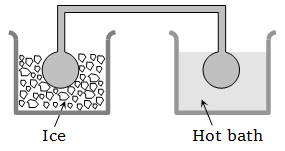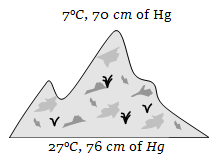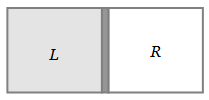The equation of state of a gas is given by $\left(\mathrm{P}+\frac{{\mathrm{aT}}^{2}}{\mathrm{V}}\right){\mathrm{V}}^{\mathrm{c}}=\left(\mathrm{RT}+\mathrm{b}\right),$ where a, b, c and R are constants. The isotherms can be represented by $\mathrm{P}={\mathrm{AV}}^{\mathrm{m}}-{\mathrm{BV}}^{\mathrm{n}},$ where A and B depend only on temperature and

1.

2.

3.

4.

High Yielding Test Series + Question Bank - NEET 2020

Difficulty Level:

70 calories of heat is required to raise the temperature of 2 moles of an ideal gas at constant pressure from 30°C to 35°C. The amount of heat required to raise the temperature of same gas through the same range (30°C to 35°C) at constant volume (R = 2 cal/mol/K)

1.  30 cal

2.  50 cal

3.  70 cal

4.  90 cal

High Yielding Test Series + Question Bank - NEET 2020

Difficulty Level:

A closed compartment containing gas is moving with some acceleration in horizontal direction. Neglect effect of gravity. Then the pressure in the compartment is

1. Same everywhere

2. Lower in the front side

3. Lower in the rear side

4. Lower in the upper side

High Yielding Test Series + Question Bank - NEET 2020

Difficulty Level:

Three closed vessels A, B and C are at the same temperature T and contain gases which obey the Maxwellian distribution of velocities. Vessel A contains only  only ${\mathrm{N}}_{2}$ and C a mixture of equal quantities of ${\mathrm{O}}_{2}$ and ${\mathrm{N}}_{2}$. If the average speed of the ${\mathrm{O}}_{2}$ molecules in vessel A is  that of the ${\mathrm{N}}_{2}$ molecules in vessel B is  the average speed of the ${\mathrm{O}}_{2}$ molecules in vessel C is

1. $\left({\mathrm{V}}_{1}+{\mathrm{V}}_{2}\right)/2$

2. ${\mathrm{V}}_{1}$

3. ${\left({\mathrm{V}}_{1}{\mathrm{V}}_{2}\right)}^{1/2}$

4.

High Yielding Test Series + Question Bank - NEET 2020

Difficulty Level:

A box containing N molecules of a perfect gas at temperature ${\mathrm{T}}_{1}$ and pressure ${\mathrm{P}}_{1}$. The number of molecules in the box is doubled keeping the total kinetic energy of the gas same as before. If the new pressure is ${\mathrm{P}}_{2}$ and temperature ${\mathrm{T}}_{2}$, then

1.

2.

3.

4.

High Yielding Test Series + Question Bank - NEET 2020

Difficulty Level:

Two identical glass bulbs are interconnected by a thin glass tube. A gas is filled in these bulbs at N.T.P. If one bulb is placed in ice and another bulb is placed in hot bath, then the pressure of the gas becomes 1.5 times. The temperature of hot bath will be1.  100°C

2.  182°C

3.  256°C

4.  546°C

High Yielding Test Series + Question Bank - NEET 2020

Difficulty Level:

Two containers of equal volume contain the same gas at pressures ${\mathrm{P}}_{1}$ and ${\mathrm{P}}_{2}$ and absolute temperatures ${\mathrm{T}}_{1}$ and ${\mathrm{T}}_{2}$ respectively. On joining the vessels, the gas reaches a common pressure P and common temperature T. The ratio P/T is equal to

1. $\frac{{\mathrm{P}}_{1}}{{\mathrm{T}}_{1}}+\frac{{\mathrm{P}}_{2}}{{\mathrm{T}}_{2}}$

2. $\frac{{\mathrm{P}}_{1}{\mathrm{T}}_{1}+{\mathrm{P}}_{2}{\mathrm{T}}_{2}}{{\left({\mathrm{T}}_{1}+{\mathrm{T}}_{2}\right)}^{2}}$

3. $\frac{{\mathrm{P}}_{1}{\mathrm{T}}_{2}+{\mathrm{P}}_{2}{\mathrm{T}}_{1}}{{\left({\mathrm{T}}_{1}+{\mathrm{T}}_{2}\right)}^{2}}$

4. $\frac{{\mathrm{P}}_{1}}{2{\mathrm{T}}_{1}}+\frac{{\mathrm{P}}_{2}}{2{\mathrm{T}}_{2}}$

High Yielding Test Series + Question Bank - NEET 2020

Difficulty Level:

At the top of a mountain a thermometer reads 7°C and a barometer reads 70 cm of Hg. At the bottom of the mountain these read 27°C and 76 cm of Hg respectively. Comparison of density of air at the top with that of bottom is1.  75/76

2.  70/76

3.  76/75

4.  76/70

High Yielding Test Series + Question Bank - NEET 2020

Difficulty Level:

The root mean square speed of the molecules of a diatomic gas is v. When the temperature is doubled, the molecules dissociate into two atoms. The new root mean square speed of the atom is

1.  $\sqrt{2}\mathrm{v}$

2.  $\mathrm{v}$

3.  $2\mathrm{v}$

4.  $4\mathrm{v}$

High Yielding Test Series + Question Bank - NEET 2020

Difficulty Level:

A vessel is partitioned in two equal halves by a fixed diathermic separator. Two different ideal gases are filled in left (L) and right (R) halves. The rms speed of the molecules in L part is equal to the mean speed of molecules in the R part. Then the ratio of the mass of a molecule in L part to that of a molecule in R part is1. $\sqrt{\frac{3}{2}}$

2. $\sqrt{\frac{\mathrm{\pi }}{4}}$

3. $\sqrt{\frac{2}{3}}$

4. $\frac{3\mathrm{\pi }}{8}$